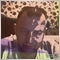• Información general
• Comentarios (1)
• Discusión (1)

# CCI Global

### Description

A universal indicator CCI together with moving averages based on it. Depending on the need, moving averages can be disabled.

You can color the indicator lines and levels: lines of CCI, moving average lines and thresholds of overbought and oversold levels.

The indicator can be calculated as the standard one on the basis of a price or as a derivative on the basis of the following eighteen other indicators included in the standard package of the MetaTrader 4 terminal:

• AD - an indicator of accumulation and distribution of volume.
• OBV - On Balance Volume.
• Volume - tick volume indicator.
• AC - indicator of acceleration.
• AO - Williams' oscillator.
• BullsPower.
• BearsPower.
• BullsPower-BearsPower - the difference of the bulls power and bears power.
• Force - Force Index indicator.
• MFI - Money Flow Index.
• ATR - Average True Range.
• Momentum.
• Moving Average.
• RSI - Relative Strength Index.
• ADX - Average Directional Movement indicator.
• DeMarker.
• Standard Deviation.
• OsMA - moving average of oscillator.
• MACD - moving average convergence/divergence.

Price is used by default.

### Purpose

The indicator can be used for manual or automated trading in an Expert Advisor. Values of indicator buffers of the double type can be used for automated trading:

1. The CCI line - buffer 0.
2. Fast MA line - buffer 1.
3. Slow MA line - buffer 2.

### Inputs

1. Calc_Method - the indicator, based on which the CCI will be calculated.
2. CCI_Period - the period of the CCI indicator.
3. Periods - the period for calculating the basic indicator, based on which CCI will be calculated.
4. FastPeriod - the fast MA period of the basic indicator (for example MACD), based on which the CCI will be calculated.
5. SlowPeriod - the slow MA period of the basic indicator.
6. SignalPeriod - the signal line period of the basic indicator.
7. MA_Method - averaging method for the MA of the basic indicator and for MAs.
8. 8. Applied_Price - the used price of the basic indicator.
9. Mode - the index of the line (MAIN or SIGNAL) of the basic indicator, based on which the CCI will be calculated.
10. Shift - the sift o the basic indicator relative to the price chart.
11. FastMA_Draw - the criterion of using in calculations and drawing the fast MA.
12. SlowMA_Draw - the criterion of using in calculations and drawing the slow MA.1917
2014.08.24 13:30

Реально отличный индикатор. Синергия в чистом виде.

Автору большое спасибо.

Versión 1.22 2020.03.27
Updating
Versión 1.21 2014.10.23
User's interface has been improved.